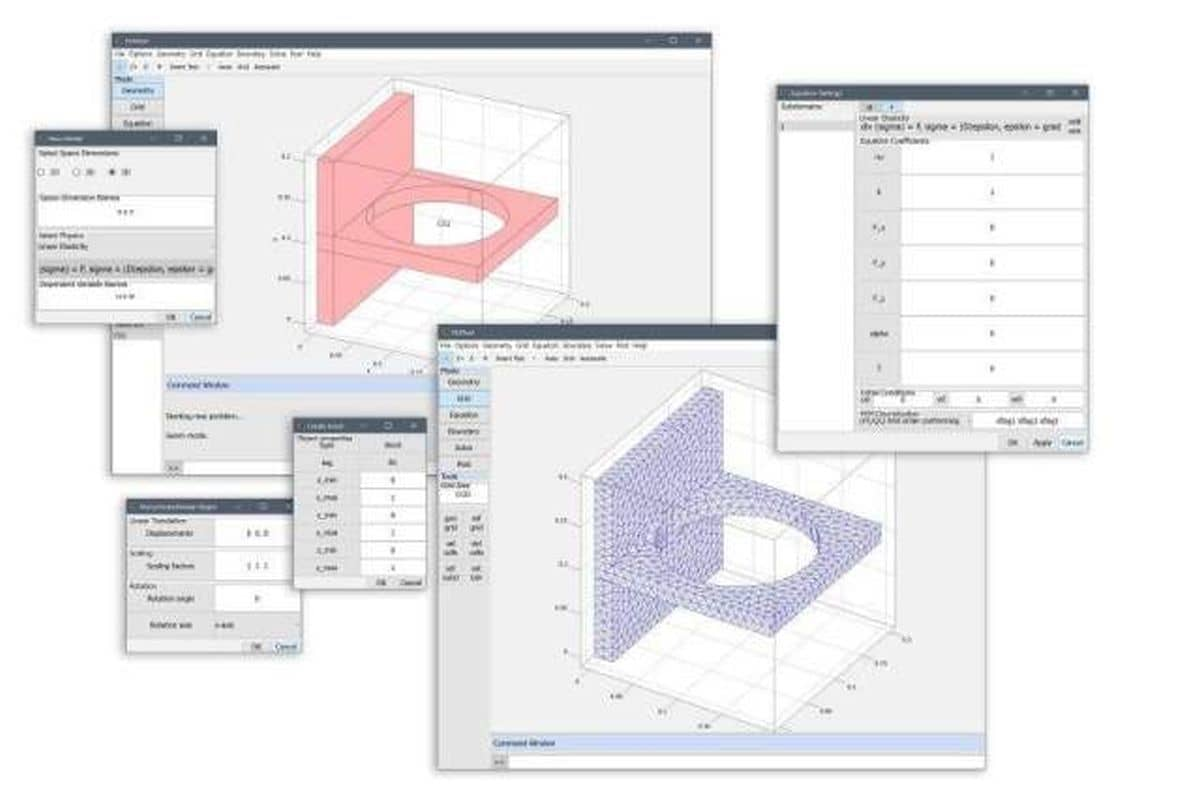# FEATool Multiphysics - Matlab Finite Element FEM Physics Simulation Toolbox

• • Fully Compatible with both Matlab and Octave
• • Easy to Use GUI and Matlab Scripting Functionality
• • Integrated CAD Geometry, Grid, Solvers, and Postprocessing Tools
• • User Customizable and Extensible## Matlab Finite Element Analysis FEM Multiphysics Toolbox

FEATool Multiphysics is an GNU Octave and Matlab FEM and PDE toolbox for modeling and simulation of physics, continuum mechanics, and engineering applications with the finite element method.

With an easy to use GUI and full suite of functions and subroutines for geometry preprocessing (CAD), grid and mesh generation, solvers, postprocessing and visualization, FEATool is a fully integrated, complete, and self contained FEM toolbox.

## Intuitive and Easy to Use GUI

FEATool features an easy to use graphical user interface (GUI) allowing full access to functionality such as geometry and CAD tools, grid generation, defining equations and multiphysics couplings, FEM assembly and solvers, as well as postprocessing.

Moreover, FEATool FEM models can be saved and exported as both compact binary data, and also Matlab m-script text file formats, the latter allowing for a fast and easy approach to learn the FEM function syntax and interfacing with other Matlab toolboxes and functions.## Predefined Multiphysics Modes

15 predefined physics modes are available which easily can be combined to set up of complex coupled multiphysics simulation problems including fluid dynamics (CFD), heat transfer, structural mechanics (SME), electromagnetics, and convective, diffusive, and reaction transport of mass and chemical species. Moreover, in FEATool editing and entering general expressions, custom equations, and PDEs is easy with the simple PDE formulation syntax.

## Customizable and Extensible

FEATool is entirely written in fully documented Octave and Matlab m-script source code, and is designed to be very easy to understand and learn. Moreover, the open approach makes the code base extensible, adaptable, and customizable to each users needs, with functionality to write Matlab m-script user defined functions, and interface with external simulation and software tools such asFeatFlowFEniCS, and Plotly.## Unique Functionality

• Multiplatform for both Octave and Matlab
• Easy to use with both GUI and CLI interface
• Matlab m-file and Python (with FEniCS) scripting
• Binary and m-file model output formats
• Easy syntax for custom PDE equations and expressions
• Built-in interfaces for external solvers
- Computational fluid dynamics CFD solver (FeatFlow)
- FEniCS multiphysics solver interface
• Web and online visualization and sharing with Plotly

## FEATool Multiphysics Features

• FEM simulation toolbox for Matlab and Octave
• Easy to use graphical user interface (GUI)
• Command line CLI scripting functionality
• 15 Predefined physics modes
- heat transfer
- fluid dynamics (CFD)
- mass transport
- structural mechanics
- electrostatics
- classic PDE equations
• 75 Tutorial and example multiphysics models
• CAD and geometry modeling in 1D, 2D, and 3D
• Supports 1D line, 2D triangular and quadrilateral, and 3D tetrahedral and hexahedral grid cells
• Automatic grid generation with simplex (line, triangle, and tetrahedral) grid cells
• Constant, 1st - 5th higher order conforming, non-conforming, and Hermite FEM basis functions
• Built-in stationary, time-dependent, linear, and non-linear solvers